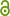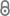# Revealing the intrinsic geometry of finite dimensional invariant sets of infinite dimensional dynamical systems

R. Gerlach, P. Koltai, M. Dellnitz, ArXiv:1902.08824 (2019).https://arxiv.org/abs/1902.08824
Preprint | English
Author
Gerlach, RaphaelLibreCat; Koltai, Péter; Dellnitz, Michael
Department
Abstract
Embedding techniques allow the approximations of finite dimensional attractors and manifolds of infinite dimensional dynamical systems via subdivision and continuation methods. These approximations give a topological one-to-one image of the original set. In order to additionally reveal their geometry we use diffusion mapst o find intrinsic coordinates. We illustrate our results on the unstable manifold of the one-dimensional Kuramoto--Sivashinsky equation, as well as for the attractor of the Mackey-Glass delay differential equation.
Publishing Year
Journal Title
arXiv:1902.08824
LibreCat-ID

### Cite this

Gerlach R, Koltai P, Dellnitz M. Revealing the intrinsic geometry of finite dimensional invariant sets of  infinite dimensional dynamical systems. arXiv:190208824. Published online 2019.
Gerlach, R., Koltai, P., & Dellnitz, M. (2019). Revealing the intrinsic geometry of finite dimensional invariant sets of  infinite dimensional dynamical systems. In arXiv:1902.08824.
@article{Gerlach_Koltai_Dellnitz_2019, title={Revealing the intrinsic geometry of finite dimensional invariant sets of  infinite dimensional dynamical systems}, journal={arXiv:1902.08824}, author={Gerlach, Raphael and Koltai, Péter and Dellnitz, Michael}, year={2019} }
Gerlach, Raphael, Péter Koltai, and Michael Dellnitz. “Revealing the Intrinsic Geometry of Finite Dimensional Invariant Sets of  Infinite Dimensional Dynamical Systems.” ArXiv:1902.08824, 2019.
R. Gerlach, P. Koltai, and M. Dellnitz, “Revealing the intrinsic geometry of finite dimensional invariant sets of  infinite dimensional dynamical systems,” arXiv:1902.08824. 2019.
Gerlach, Raphael, et al. “Revealing the Intrinsic Geometry of Finite Dimensional Invariant Sets of  Infinite Dimensional Dynamical Systems.” ArXiv:1902.08824, 2019.
All files available under the following license(s):Closed Access